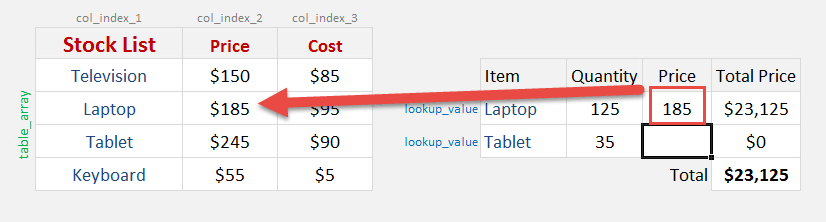What does it do?

Searches for a value in the first column of a table array and returns a value in the same row from another column (to the right) in the table array.

Formula breakdown:

=VLOOKUP(lookup_value, table_array, col_index_num, [range_lookup])

What it means:

=VLOOKUP(this value, in this Named Range, and get me value in this column, Exact Match/FALSE/0])

A Named Range makes it easier to understand Excel formulas, especially if the said formula contains an array argument.

A Named Range can be a cell, a cell range, a Table, a function, or a constant.STEP 1: To define a Named Range in Excel you need to select the cell/cell range/Table/function/constant and go to the Name Box which is located on the top left-hand corner of the workbook – next to the Formula Bar.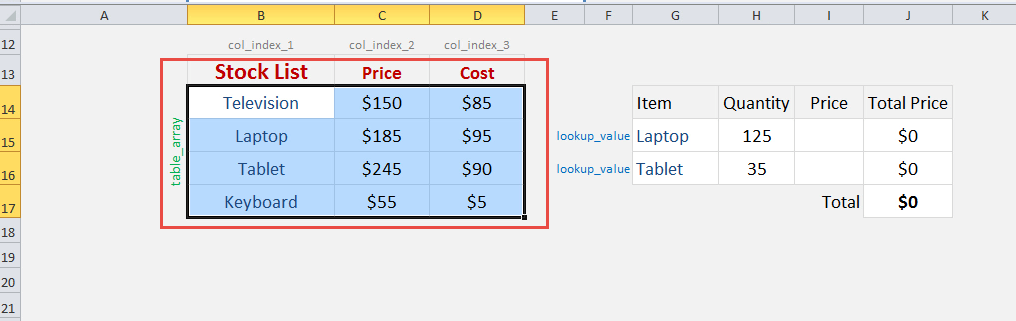STEP 2: In here you can name your range whatever you like (make sure there are no spaces) and press Enter.  You can view your Named Range by clicking on the drop-down box in the Name Box.  In our example, we will give this a name of StockList.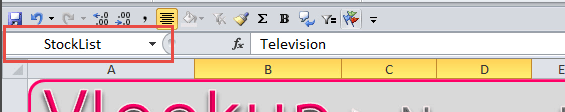You can also view/edit/delete your Named Range by going to the Formulas tab in the Ribbon menu and selecting Name Manager.

STEP 3: Now that you are all set, each time you are creating a formula, like a Vlookup formula, it is best to use a Named Range as it makes the formula easier to understand and maintain.

We need to enter the Vlookup function:

## =VLOOKUP(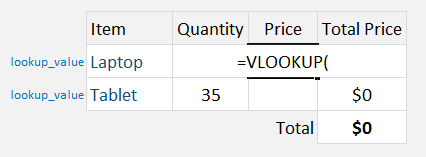The Vlookup arguments:

## lookup_value

What are we looking for?

Reference the cell that contains the text or value:

## =VLOOKUP(G15,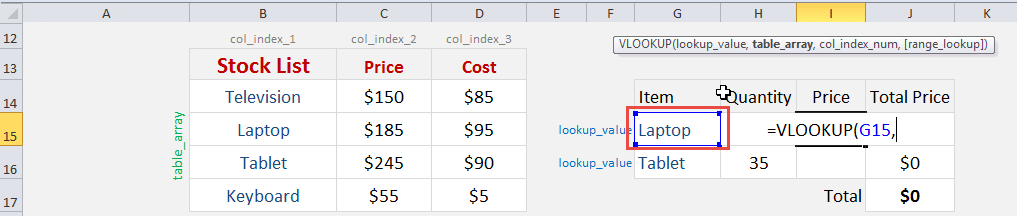## table_array

From which list are we doing a lookup on?

The formula for Excel VlookUp Named Range will be:

## =VLOOKUP(G15, StockList,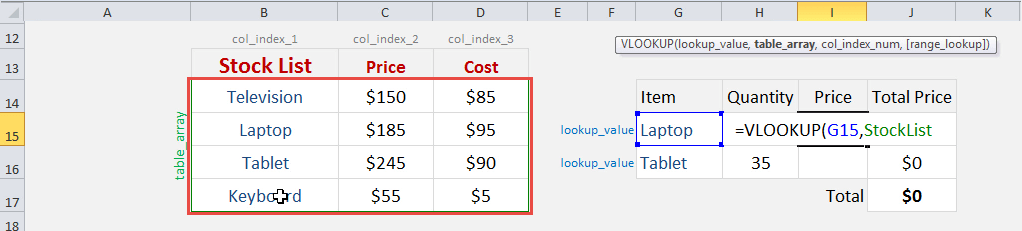## col_index_num

From which column do we want to retrieve the value?

We want to retrieve the Price which is the SECOND column from our table array:

## =VLOOKUP(G15, StockList, 2,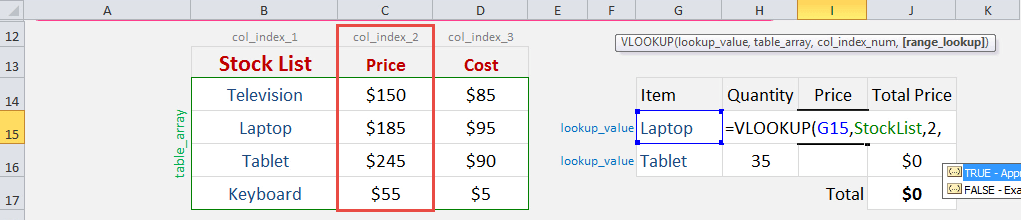## [range_lookup]

Do we want an exact match?

Place in FALSE to signify that we want an exact match:

## =VLOOKUP(G15, StockList, 2, FALSE)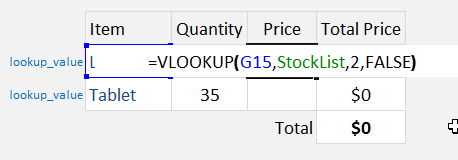This is how the price will now dynamically change based on your selection with Vlookup using named range: